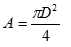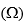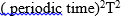##### Search### KCSE CLUSTER TESTS 25

#### Physics Paper 3

1.

1. You are provided with the following

-Voltmeter (0-5V)
-Resistance wire 1m.

-A cell and a cell holder

-Ammeter (0-1.25A)

-Micrometer screw gauge.

-Six connecting wires.

-Three crocodile clips

-One metre long Nichrome wire fixed on the bench

-Half metre rule or metre rule.

Proceed as follows

a) Using a micrometer screw gauge i) Measure diameter D of the MN

D…………………………………………………………………………………

ii) Determine area A, Given thatb) i) Connect the apparatus provided as shown in the circuit diagram below, MN is the Nichrome wire.Leave the crocodile clip next to the cell connected. This clip should be connected when no readings are being taken.

ii) With L set at 80cm, record the value of ammeter and voltmeter reading in the table below.

iii) Repeat step (ii) by moving crocodile clip such that L=60 40 30 20 and 10cm.c) i) On the grid provided plot a graph of Resistanceagainst L(m)(ii) Determine the slope m of the graph

(iii) Given the20 marks

2.

You are provided with the following:

2 retort stands and 3 clamps.

1 metre rule.

1 brick.

1 spiral spring with a pointer.

1 metre rule

One 50g mass

Five 20g mass

A stop watch

Procedure

a) Arrange the apparatus as shown below.(make sure the retort stand is firm by putting a brick on the base).b) Use the second retort stand to fit a metre rule vertically in such a way that the pointer is horizontal across the scale.

c) Note the position of the pointer.

d) Add a 50g mass to the hanger on the spring and note the new pointer reading. Determine the extension of the spring.

e) Set the spring and mass into vertical oscillation with small amplitude and record the time t, for 50 oscillations.

f) Calculate the periodic time, T, and record in the table.

g) Repeat (b),(c),(d),(e) and f above for 70g,90g,110g,130g and 150g masses.

h) Determine T2 in each case and complete the table below.I. Plot a graph of extension of spring against theII. Determine the gradient of the graph

III. The graph is given by the equationDetermine the value of Q.

…………………………………………………………………………………………………

…………………………………………………………………………………………………

IV. What is the significance of Q?

…………………………………………………………………………………………………

…………………………………………………………………………………………………

20 marks

Back Top